## NCERT Solutions for Class 6 Maths Chapter 3 Playing With Numbers

Free download NCERT Solutions for Class 6 Maths Chapter 3 Exercise 3.1, Ex 3.2, Ex 3.3, Ex 3.4, Ex 3.5, Ex 3.6 and Ex 3.7 Playing with Numbers PDF for CBSE 2020 Exams.

### Playing with Numbers Class 6 Ex 3.1

Ex 3.1 Class 6 Maths Question 1.
Write all the factors of the following numbers:
(a) 24 (b) 15 (c) 21
(d) 27 (e) 12 (f) 20
(g) 18 (h) 23 (i) 36
Solution:
(a) Factors of 24 are:
24 = 1 x 24;
24 = 2 x 12;
24 = 3 x 8;
24 = 4 x 6
Hence, all the factors of 24 are: 1, 2, 3, 4, 6, 8, 12 and 24.

(b) Factors of 15 are:
15 = 1 x 15;
15 = 3 x 5
Hence, all the factors of 15 are: 1, 3, 5 and 15.

(c) Factors of 21 are:
21 = 1 x 21;
21 = 3 x 7
Hence, all the factors of 21 are: 1, 3, 7 and 21.

(d) Factors of 27 are:
27 = 1 x 27;
27 = 3 x 9.
Hence, all the factors of 27 are: 1, 3, 9 and 27.

(e) Factors of 12 are:
12 = 1 x 12;
12 = 2 x 6;
12 = 3 x 4
Hence, all the factors of 12 are: 1, 2, 3, 4, 6 and 12.

(f) Factors of 20 are:
20 = 1 x 20;
20 = 2 x 10;
20 = 4 x 5
Hence, all the factors of 20 are: 1, 2, 4, 5, 10 and 20.

(g) Factors of 18 are:
18 = 1 x 18;
18 = 2 x 9;
18 = 3 x 6
Hence, all the factors of 18 are: 1, 2, 3, 6, 9 and 18.

(h) Factors of 23 are:
23 = 1 x 23
Hence, all the factors of prime number 23 are: 1 and 23.

(i) Factors of 36 are:
36 = 1 x 36;
36 = 2 x 18;
36 = 3 x 12;
36 = 4 x 9;
36 = 6 x 6
Hence, all the factors of 36 are: 1, 2, 3, 4, 6, 9, 12, 18 and 36.

Ex 3.1 Class 6 Maths Question 2.
Write first five multiples of:
(a) 5 (b) 8 (c) 9
Solution:
(a) First five multiples of 5 are:
5 x 1 = 5;
5 x 2 = 10;
5 x 3 = 15;
5 x 4 = 20;
5 x 5 = 25
Hence, the required multiples of 5 are: 5, 10, 15, 20 and 25.

(b) First five multiples of 8 are:
8 x 1 = 8;
8 x 2 = 16;
8 x 3 = 24;
8×4 = 32;
8 x 5 = 40
Hence, the required multiples of 8 are: 8, 16, 24, 32 and 40.

(c) First five multiples of 9 are:
9 x 1 = 9;
9 x 2 = 18;
9 x 3 = 27;
9 x 4 = 36;
9 x 5 = 45
Hence, the required multiples of 9 are: 9,18, 27, 36 and 45.

Ex 3.1 Class 6 Maths Question 3.
Match the items in column I with the items in column II.Solution:
(i) ↔ (b) [∵ 7 x 5 = 35]
(ii) ↔ (d) [∵ 15 x 2 = 30]
(iii) ↔ (a) [∵ 8 x 2 = 16]
(iv) ↔ (f) [∵ 20 x 1 = 20]
(v) ↔ (e) [∵ 25 x 2 = 50]

Ex 3.1 Class 6 Maths Question 4.
Find all the multiples of 9 upto 100.
Solution:
9 x 1 = 9;
9 x 2 = 18;
9 x 3 = 27;
9 x 4 = 36;
9 x 5 = 45;
9 x 6 = 54;
9 x 7 = 63;
9 x 8 = 72;
9 x 9 = 81;
9 x 10 = 90;
9 x 11 = 99
Hence, all the multiples of 9 upto 100 are:
9, 18, 27, 36, 45, 54, 63, 72, 81, 90 and 99.

Move to Top → Playing with Numbers

### Playing with Numbers Class 6 Ex 3.2

Ex 3.2 Class 6 Maths Question 1.
What is the sum of any two:
(a) Odd numbers?
(b) Even numbers?
Solution:
(a) The sum of any two odd numbers is even.
(b) The sum of any two even numbers is even.

Ex 3.2 Class 6 Maths Question 2.
State whether the following statements are True or False.
(a) The sum of three odd numbers is even.
(b) The sum of two odd numbers and one even number is even.
(c) The product of three odd numbers is odd.
(d) If an even number is divided by 2, the quotient is always odd.
(e) All prime numbers are odd.
(f) Prime numbers do not have any factors.
(g) Sum of two prime numbers is always even.
(h) 2 is only the even prime number.
(i) All even numbers are composite numbers.
(j) The product of any two even numbers is always even.
Solution:
(а) False [∵ 3 + 5 + 7 = 15 (odd)]
(b) True [∵ 3 + 5 + 6 = 14 (even)]
(c) True [∵ 5 x 7 x 9 = 315 (odd)]
(d) False [∵ 6 + 2 = 3 (odd)]
(e) False [∵ 2 is a prime number but it is even]
(f) False [∵ 3 is a prime number having 1 and 3 as its factors]
(g) False [∵ 7 + 2 = 9 (odd)]
(h) True [∵ 2 is even and the lowest prime number]
(i) False [∵ 2 is even but not composite number]
(j) True [∵ 4 x 6 = 24 (even)]

Ex 3.2 Class 6 Maths Question 3.
The numbers 13 and 31 are prime numbers. Both these numbers have same digits 1 and 3. Find such pairs of prime numbers up to 100.
Solution:
The required pair of prime numbers having same digits are:
(17 and 71), (37 and 73), (79 and 97).

Ex 3.2 Class 6 Maths Question 4.
Write down separately the prime and composite numbers less than 20.
Solution:
Prime numbers less than 20 are:
2, 3, 5, 7, 11, 13, 17 and 19
Composite numbers less than 20 are:
4, 6, 8, 9, 10, 12, 14, 15, 16 and 18

Ex 3.2 Class 6 Maths Question 5.
What is the greatest prime number between 1 and 10?
Solution:
The greatest prime number between 1 and 10 is 7.

Ex 3.2 Class 6 Maths Question 6.
Express the following as the sum of two odd primes.
(a) 44
(b) 36
(c) 24
(d) 18
Solution:
(a) 44 = 13 + 31
(b) 36 = 17 + 19
(c) 24 = 7 + 17
(d) 18 = 7 + 11

Ex 3.2 Class 6 Maths Question 7.
Give three pairs of prime numbers whose difference is 2.
[Remark: Two prime numbers whose difference is 2 are called twin primes]
Solution:
Required pairs are: (3 and 5), (5 and 7) and (11 and 13)

Ex 3.2 Class 6 Maths Question 8.
Which of the following numbers are prime?
(a) 23
(b) 51
(c) 37
(d) 26
Solution:
(a) 23 is a prime number [ ∵ 23 = 1 x 23]
(b) 51 is not a prime number [∵ 51 = 1 x 3 x 17]
(c) 37 is a prime number [∵ 37 = 1 x 37]
(d) 26 is not a prime number [∵ 26 = 1 x 2 x 13]

Ex 3.2 Class 6 Maths Question 9.
Write seven consecutive composite numbers less than 100 so that there is no prime number between them.
Solution:
Required seven consecutive composite numbers are:
90, 91, 92, 93, 94, 95 and 96

Ex 3.2 Class 6 Maths Question 10.
Express each of the following numbers as the sum of three odd primes.
(a) 21
(b) 31
(c) 53
(d) 61
Solution:
(a) 21 can be expressed as 3 + 5 + 13
(b) 31 can be expressed as 5 + 7 + 19
(c) 53 can be expressed as 13 + 17 + 23
(d) 61 can be expressed as 11 + 13 + 37

Ex 3.2 Class 6 Maths Question 11.
Write five pairs of prime numbers less than 20 whose sum is divisible by 5.
(Hint: 3 + 7 = 10)
Solution:
Required pairs of prime numbers less than 20 are:
(i) 2 + 3 = 5
(ii) 2 + 13 = 15
(iii) 11 + 9 = 20
(iv) 17 + 3 = 20
(v) 7 + 13 = 20

Ex 3.2 Class 6 Maths Question 12.
Fill in the blanks.
(a) A number which has only two factors is called a ………… .
(b) A number which has more than two factors is called a ………… .
(c) 1 is neither ………… nor ………… .
(d) The smallest prime number is ………… .
(e) The smallest composite number is ………… .
(f) The smallest even number is ………… .
Solution:
(a) prime number
(b) composite number
(c) prime, composite
(d) 2
(e) 4
(f) 2

Move to Top → Playing with Numbers

Move to Top → Playing with Numbers

### Playing with Numbers Class 6 Ex 3.3

Ex 3.3 Class 6 Maths Question 1.
Using divisibility tests, determine which of the following numbers are divisible by 2, by 3, by 4, by 5, by 6, by 8, by 9, by 10, by 11 (Say, Yes or No)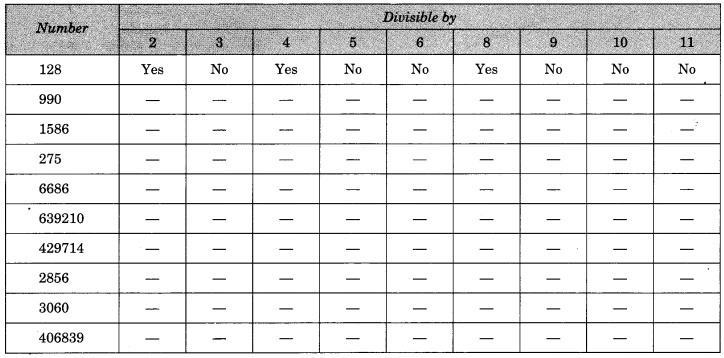Solution: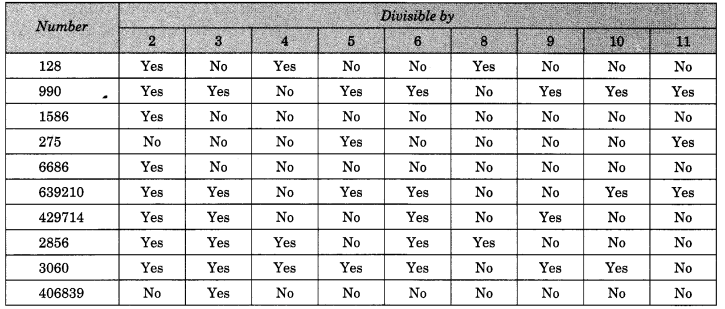Ex 3.3 Class 6 Maths Question 2.
Using divisibility tests, determine which of following numbers are divisible by 4; by 8.
(a) 572
(b) 726352
(c) 5500
(d) 6000
(e) 12159
(f) 14560
(g) 21084
(j) 2150
(h) 31795072
(i) 1700
Solution:
(a) Given number = 572
(i) Divisibility by 4
Here, the number formed by the last two digits of the given number is 72.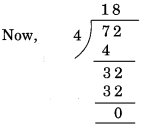Remainder 0. Hence, 572 is divisible by 4.

(ii) Divisibility by 8
Here, the number formed by the last three digits of the given number is 572.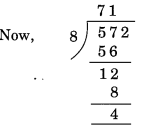Remainder 4. Hence, 572 is not divisible by 8.

(b) Given number = 726352
(i) Divisibility by 4
Here, the number formed by the last two digits of the given number = 52.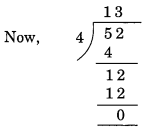Remainder = 0.
Hence, 726352 is divisible by 4.

(ii) Divisibility by 8
Here, the number formed by the last three digits of the given number = 352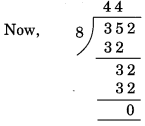Remainder = 0.
Hence, 726352 is divisible by .

(c) Given number = 5500
(i) Divisibility by 4
Here the last two digits of the given number are 0. Hence, 5500 is divisible by 4.
(ii) Divisibility by 8
Here, the number formed by the last three digits of the given number = 500Remainder = 4. Hence, 5500 is not divisible by 8.

(d) Given number = 6000
(i) Divisibility by 4
Here, the last two digits of the given number are 0.
Hence, 6000 is divisible by 4.

(ii) Divisibility by 8
Here, the last three digits of the given number are 0.
Hence, 6000 is divisible by 8.

(e) Given number = 12159
(i) Divisibility by 4
Here, the number formed by last two digits of the given number = 59Remainder = 3.
Hence, 12159 is divisible by 4.

(ii) Divisibility by 8
Here, the number formed by the last three digits of the given number = 159Remainder = 7.
Hence, 12159 is not divisible by 8.

(f) Given number = 14560
(i) Divisibility by 4
Here, the number formed by the last two digits of the given number = 60.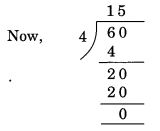Remainder = 0.
Hence, 14560 is divisible by 8.

(g) Given number = 21084
(i) Divisibility by 4
Here, the number formed by the last two digits of the given number = 84.Remainder = 0. Hence, 21084 is divisible by 4.

(ii) Divisibility by 8
Here, the number formed by the last three digits of the given number = 084.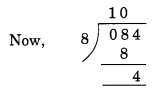Remainder = 4.
Hence, 21084 is not divisible by 8.

(h) Given number = 31795072
(i) Divisibility by 4
Here, the number formed by the last two digits of the given number = 72.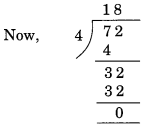Remainder = 0. Hence, 31795072 is divisible by 4.

(ii) Divisibility by 8
Here, the number formed by the last three digits of the given number = 072.Remainder = 0. Hence, 31795072 is divisible by 8.

(i) Given number = 1700
(i) Divisibility by 4
Here, the last two digits of the given number is 0. Hence, 1700 is divisible by 4.
(ii) Divisibility by 8
Here, the number formed by the last three digits of the given number = 700Remainder = 4. Hence, 1700 is not divisible by 8.
(j) Given number = 2150
(i) Divisibility by 4
Here, the number formed by the last two digits of the given number = 50.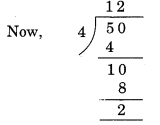Remainder = 2. Hence, 2150 is not divisible by 4.

(ii) Divisibility by 8
Here, the number formed by the last three digits of the given number = 150Remainder = 6. Hence, 2150 is not divisible by 8.

Ex 3.3 Class 6 Maths Question 3.
Using divisibility tests, determine which of the following numbers are divisible by 6:
(a) 297144
(b) 1258
(c) 4335
(d) 61233
(e) 901352
(f) 438750
(g) 1790184
(h) 12583
(i) 639210
(j) 17852
Solution:
We know that a number is divisible by 6 if it is also divisible by both 2 and 3.
(a) Given number = 297144
The given number 297144 has even digit at its ones place.
So, it is divisible by 2.
The sum of all the digits of 297144 = 2 + 9 + 7 + 1 + 4 + 4 = 27
which is divisible by 3.
Hence, the given number 297144 is divisible by 6.

(b) Given number = 1258
The given number 1258 has even digit i.e., 8 at its ones place.
So, it is divisible by 2.
The sum of all digits of 1258 = l + 2 + 5 + 8 = 16 which is not divisible by 3.
Hence, the given number 1258 is not divisible by 6.

(c) Given number = 4335
The digit at ones place of the given number is not even.
So, it is not divisible by 2.
The sum of all the digits of 4335 = 4 + 3 + 3 + 5 = 15 which is divisible by 3.
Since the given number 4335 is not divisible by both 2 and 3 therefore, it is not divisible by 6.

(d) Given number = 61233
The digit at ones place of the given number is not even.
So, it is not divisible by 2.
The sum of the digits of the given number 61233 = 6 + 1 + 2 + 3 + 3 = 15 which is divisible by 3.
Since, the given number is not divisible by both 2 and 3, it is not divisible by 6.

(e) Given number = 901352
The digit at ones place of the given number is even.
So, it is divisible by 2.
The sum of all the digits of the given number 901352 = 9 + 0 + 1 + 3 + 5 + 2 = 20 which is not divisible by 3.
Since, the given number is not divisible by both 2 and 3 hence, it is not divisible by 6.

(f) Given number = 438750
The digit at ones place of the given number is 0. So, it is divisible by 2.
The sum of all the digits of the given number 438750
=4 + 3 + 8 + 7 + 5 + 0 = 27 which is divisible by 3.
Hence, the given number is divisible by 6.

(g) Given number = 1790184
The digit at ones place of the given number is even.
So, it is divisible by 2.
The sum of all the digits of the given number 1790184
= 1 + 7 + 9 + 0 + 1 + 8 + 4 = 30 which is divisible by 3.
Hence, the given number is divisible by 6.

(h) Given number = 12583
The digit to ones place of the given number is odd.
So, it is not divisible by 2.
Sum of all the digits of the given number 12583
= 1 + 2 + 5 + 8 + 3 = 19
which is not divisible by 3.
Hence, the given number is not divisible by 6.

(i) Given number = 639210
The digit at ones place of the given number is 0.
So, it is divisible by 2.
The sum of all the digits of the given number 639210
= 6 + 3 + 9 + 2 + 1 + 0 = 21 which is divisible by 3.
Hence, the given number is divisible by 6.

(j) Given number = 17852
The digit at ones place of the given number is even.
So, it is divisible by 2.
The sum of all the digits of the given number 17852
= 1 + 7 + 8 + 5 + 2 = 23 which is not divisible by 3.
Hence, the given number is not divisible by 6.

Ex 3.3 Class 6 Maths Question 4.
Using divisibility tests, determine which of the following numbers are divisible by 11:
(a) 5445
(b) 10824
(c) 7138965
(d) 70169308
(e) 10000001.
Sol. We know that a number is divisible by 11 if the difference between the sum of the digits at odd places (from the right) and the sum of the digits at even places (from the right) of the number is either 0 or divisible by 11.
(a) Given number = 5445
Sum of the digits at odd places = 5 + 4 = 9
Sum of the digits at even places = 4 + 5 = 9
Difference = 9 – 9 = 0
Hence, the given number is divisible by 11.

(b) Given number = 10824
Sum of the digits at odd places = 4 + 8 + 1 = 13
Sum of the digits at even places = 2 + 0 = 2
Difference = 13 – 2 = 11
which is divisible by 11.
Hence, the given number is divisible by 11.

(c) Given number = 7138965
Sum of the digits at odd places = 5 + 9 + 3 + 7 = 24
Sum of the digits at even places = 6 + 8 + 1 = 15
Difference = 24 – 15 = 9
which is not divisible by 11.
Hence, the given number is not divisible by 11.

(d) Given number = 70169308
Sum of all the digits at odd places = 8 + 3 + 6 + 0 = 17
Sum of all the digits at even places = 0 + 9 + 1 + 7 = 17
Difference = 17-17 = 0
Hence, the given number is divisible by 11.

(e) Given number = 10000001
Sum of all the digits at odd places = 1 + 0 + 0 + 0 = 1
Sum of all the digits at even places = 0 + 0 + 0 + 1 = 1
Difference = 1 – 1 = 0
Hence, the given number is divisible by 11.

Ex 3.3 Class 6 Maths Question 5.
Write the smallest digit and the greatest digit in the blank space of each of the following numbers so that the number formed is divisible by 3.
(a) ____ 6724
(b) 4765 ____ 2
Solution:
We know that number is divisible by 3 if the sum of all the digits of the number is also divisible by 3.
(a) ___ 6724
Sum of the digits = 4 + 2 + 7 + 6 = 19
The smallest digit to be placed is blank space = 2
Then the sum = 19 + 2 = 21 which is divisible by 3.
The greatest digit to be placed in blank space = 8
Then, the sum = 19 + 8 = 27 which is divisible by 3
Hence, the required digits are 2 and 8.

(b) 4765 ____ 2.
Sum of digits = 2 + 5 + 6 + 7 + 4 = 24
The smallest digits to be place in blank space = 0
Then, sum = 24 + 0 = 24
which is divisible by 3.
The greatest digit to be placed in blank space = 9.
Then, the sum = 24 + 9 = 33 which is divisible by 3.
Hence, the required digits are 0 and 9.

Ex 3.3 Class 6 Maths Question 6.
Write a digit in the blank space of each of the following numbers so that the numbers formed is divisible by 11.
(a) 92 ___ 389
(b) 8 ___ 9484
Solution:
(a) 92 ___ 389
Sum of the digits at odd places = 9 + 3 + 2 = 14
Sum of the digits at even places = 8 + ( ) + 9 = 17
Difference = 17 + ( ) – 14 = () + 3
For the given number to be divisible by 11
( ) + 3 = 11
∴ ( ) = 11 – 3 = 8
So, the missing digit = 8
Hence, the required number is 928389.

(b) 8 ___ 9484
Sum of the digits at odd places = 4 + 4 + ( ) = 8 + ( )
Sum of the digits at even places = 8 + 9 + 8 = 25
∴ Difference = 25 – [8 + ( )]
= 25 – 8 – ( ) = 17 – ( )
For the given number to be divisible by 11
17 – 0 = 11
∴ 17 – 11 = 6
So, the missing digit = 6
Hence, the required number = 869484.

Move to Top → Playing with Numbers

### Playing with Numbers Class 6 Ex 3.4

Ex 3.4 Class 6 Maths Question 1.
Find the common factors of:
(a) 20 and 28
(b) 15 and 25
(c) 35 and 50
(d) 56 and 120
Solution:
(a) Given numbers are : 20 and 28
Factors of 20 are 1, 2, 4, 5, 10, 20
Factors of 28 are 1, 2, 4, 7, 28
Hence, the common factors are 1, 2 and 4.

(b) Given numbers are: 15 and 25
Factors of 15 are 1, 3, 5, 15
Factors of 25 are 1, 5, 25
Hence, the common factors are 1 and 5.

(c) Given numbers are: 35 and 50
Factors of 35 are: 1, 5, 7, 35
Factors of 50 are: 1, 2, 5, 10, 50
Hence, the common factors are 1 and 5.

(d) Given numbers are: 56 and 120
Factors of 56 are 1, 2, 4, 7, 8, 14, 28, 56
Factors of 120 are 1, 2, 3, 4, 5, 6, 8, 10, 12, 15, 20, 30, 40, 60, 120
Hence, the common factors are 1,2, 4, and 8.

Ex 3.4 Class 6 Maths Question 2.
Find the common factors of:
(a) 4, 8 and 12
(b) 5, 15 and 25
(6) 8 9484
Solution:
(a) Given numbers are: 4, 8 and 12
Factors of 4 are 1, 2, 4
Factors of 8 are 1, 2, 4, 8
Factors of 12 are 1, 2, 3, 4, 6, 12
Hence, the common factors are 1, 2 and 4.

(b) Given numbers are: 5, 15 and 25
Factors of 5 are 1, 5
Factors of 15 are 1, 3, 5, 15
Factors of 25 are 1, 5, 25
Hence, the common factors are 1 and 5.

Ex 3.4 Class 6 Maths Question 3.
Find first three multiples of:
(a) 6 and 8
(b) 12 and 18
Solution:
(a) Given numbers are 6 and 8
First three multiples of 6 are
6 x 1= 6; 6 x 2 = 12; 6 x 3 = 18.
First three multiples of 8 are
8 x 1 = 8; 8 x 2 = 16; 8 x 3 = 24.

(b) Given numbers are 12 and 18.
First three multiples of 12 are
12 x 1 = 12;
12 x 2 = 24;
12 x 1 = 36;
First three multiples of 18 are
18 x 1 = 18;
18 x 2 = 36;
18 x 3 = 54.

Ex 3.4 Class 6 Maths Question 4.
Write all the numbers less than 100 which are common multiples of 3 and 4.
Solution:
Given numbers are 3 and 4.
Multiples of 3 less than 100 are:Hence, the common multiples of 3 and 4 less than 100 are: 12, 24, 36, 48, 60, 72, 84 and 96.

Ex 3.4 Class 6 Maths Question 5.
Which of the following numbers are co-prime?
(a) 18 and 35
(b) 15 and 37
(c) 30 and 415
(d) 17 and 68
(e) 216 and 215
(f) 81 and 16
Solution:
(a) Given number are 18 and 35
Factors of 18 are 1, 2, 3, 6, 9, 18
Factors of 35 are 1, 5, 7, 35
Since, the common factors of 18 and 35 is only 1.
Hence, 18 and 35 are co-prime.

(b) Given numbers are 15 and 37
Factors of 15 are 1, 3, 5, 15
Factors of 37 are 1,37
Since, the common factor of 15 and 37 is only 1.
Hence, they are co-prime.

(c) Given numbers are 30 and 415
Factors of 30 are 1, 2, 3, 5, 6, 15, 30
Factors of 415 are 1, 5, 83
Since, the numbers have common factors 1 and 5
Hence, they are not co-prime.

(d) Given numbers are 17 and 68
Factors of 17 are 1, 17
Factors of 68 are 1, 2, 4, 17, 34, 68
Since, the numbers have common factors 1 and 17
Hence, they are not co-prime.

(e) Given numbers are 216 and 215
Factors of 216 are 1, 2, 3, 4, 6, 8, 9, 12, 18, 24, 36, 54, 72, 108, 216
Factors of 215 are 1, 5, 43
Since only 1 is the common factor of 216 and 215.
Hence, they are co-prime.

(f) Given numbers are 81 and 16
Factors of 81 are 1, 3, 9, 27, 81
Factors of 16 are 1, 2, 4, 8, 16
Since only 1 is common to 81 and 16
Hence, they are co-prime.

Ex 3.4 Class 6 Maths Question 6.
A number is divisible by both 5 and 12. By which other will that number be always divisible?
Solution:
If the number is divisible by both 5 and 12 this the number will also be divisible by 5 x 12 i.e., 60.

Ex 3.4 Class 6 Maths Question 7.
A number is divisible by 12. By what other will that number be divisible?
Solution:
Factors of 12 are 1, 2, 3, 4, 6, 12
Hence the number which is divisible by 12, will also be divisible by its factors i.e., 1, 2, 3, 4, 6 and 12.

Move to Top → Playing with Numbers

### Playing with Numbers Class 6 Ex 3.5

Ex 3.5 Class 6 Maths Question 1.
Which of the following statements are true?
(a) If a number is divisible by 3, it must be divisible by 9.
(b) If a number is divisible by 9, it must be divisible by 3.
(c) A number is divisible by 18, if it is divisible by both 3 and 6.
(d) If a number is divisible by 9 and 10 both, then it must be divisible by 90.
(e) If two numbers are co-primes, at least one of them must be prime.
(f) All numbers which are divisible by 4 must also be divisible by 8.
(g) All numbers which are divisible by 8 must also be divisible by 4.
(h) If a number exactly divides two numbers separately,- it must exactly divide their sum.
(i) If a number exactly divides the sum of two numbers, it must exactly divide the two numbers separately.
Solution:
(a) False
(b) True
(c) False
(d) True
(e) False
(f) False
(g) True
(h) True
(i) False

Ex 3.4 Class 6 Maths Question 2.
Here are two different factor trees for 60. Write the missing numbers.Solution:
Given thatHere, 6 = 2 x missing number
∴ Missing number = 6 2 = 3
Similarly, 10 = 5 x missing number
∴ Missing number = 10 ÷ 5 = 2
Hence, the missing numbers are 3 and 2.

(b) Given that: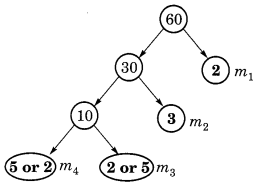Let the missing numbers be m1, m2, m3 and m4.
60 = 30 x m1
⇒ m1 = 60 ÷ 30 = 2
30 = 10 x m2
⇒ m2 = 30 ÷ 10 = 3
10 = m3 x m4
⇒ m3 = 2 or 5 and m4 = 5 or 2
Hence, the missing numbers are 2, 3, 2, 5.

Ex 3.4 Class 6 Maths Question 3.
Which factors are not included in the prime factorisation of a composite number?
Solution:
1 and the number itself are not included in the prime factorisation of a composite number.

Ex 3.4 Class 6 Maths Question 4.
Write the greatest 4-digit number and express it in terms of its prime factors.
Solution:
The greatest 4-digit number = 9999Hence, the prime factors of 9999 = 3 x 3 x 11 x 101.

Ex 3.4 Class 6 Maths Question 5.
Write the smallest 5-digit number and express it in the form of its prime factors.
Solution:
The smallest 5-digit number = 10000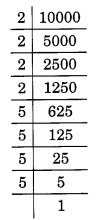Hence, the required prime factors: 10000 = 2 x 2 x 2 x 2 x 5 x 5 x 5 x 5.

Ex 3.4 Class 6 Maths Question 6.
Find all the prime factors of 1729 and arrange them in ascending order. Now state the relations, if any, between the two consecutive prime factors.
Solution:
Given number = 1729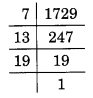Hence, the prime factors of 1729 = 7 x 13 x 19.
Here, 13 – 7 = 6 and 19 – 13 = 6
We see that the difference between two consecutive prime factors is 6.

Ex 3.4 Class 6 Maths Question 7.
The product of three consecutive numbers is always divisible by 6. Verify this statement with the help of some examples.
Solution:
Example 1:
Take three consecutive numbers 20, 21 and 22.
Here 20 is divisible by 2 and 21 is divisible by 3.
Therefore, the product 20 x 21 x 22 = 9240 is divisible by 6.

Example 2:
Take three consecutive numbers 30; 31 and 32.
Here 30 is divisible by 3 and 32 is divisible by 2.
Therefore, the product 30 x 31 x 32 = 29760 is divisible by 6.

Example 3:
Take three consecutive numbers 48, 49 and 50.
Here, 48 is divisible by 3 and 50 is divisible by 2.
Therefore, the product 48 x 49 x 50 = 117600 which is divisible by 6.

Ex 3.4 Class 6 Maths Question 8.
The sum of two consecutive odd numbers is divisible by 4. Verify this statement with the help of some examples.
Solution:
Example 1:
Let us take two consecutive odd numbers 97 and 99.
Sum = 97 + 99 = 196
Here, the number formed by last two digits is 96 which is divisible by 4.
Hence, the sum of numbers 97 and 99 i.e. 196 is divisible by 4.

Example 2:
Let us take two consecutive odd numbers 121 and 123.
Sum = 121 + 123 = 244
Here, the number formed by last two digits is 44 which is divisible by 4.

Example 3:
Let us take two consecutive odd numbers 105 and 107.
Sum = 105 + 107 = 212
Here, the number formed by the last two digits is 12 which is divisible by 4.

Ex 3.4 Class 6 Maths Question 9.
In which of the following expressions, prime factorisation has been done?
(a) 24 = 2 x 3 x 4
(b) 56 = 7 x 2 x 2 x 2
(c) 70 = 2 x 5 x 7
(d) 54 = 2 x 3 x 9.
Solution:
(a) 24 = 2 x 3 x 4
Here, 4 is not a prime number.
Hence, 24 = 2 x 3 x 4 is not a prime factorisation.

(b) 56 = 7 x 2 x 2 x 2
Here, all factors are prime numbers
Hence, 56 = 7 x 2 x 2 x 2 is a prime factorisation.

(c) 70 = 2 x 5 x 7
Here, all factors are prime numbers.
Hence, 70 = 2 x 5 x 7 is a prime factorisation.

(d) 54 = 2 x 3 x 9
Here, 9 is not a prime number.
Hence, 54 = 2 x 3 x 9 is not a prime factorisation.

Ex 3.4 Class 6 Maths Question 10.
Determine if 25110 is divisible by 45.
Solution:
45 = 5 x 9
Here, 5 and 9 are co-prime numbers.
Test of divisibility by 5: unit place of the given number 25110 is 0. So, it is divisible by 5.
Test of divisibility by 9:
Sum of the digits = 2 + 5 + l + l + 0 = 9 which is divisible by 9.
So, the given number is divisible by 5 and 9 both. Hence, the number 25110 is divisible by 45.

Ex 3.4 Class 6 Maths Question 11.
18 is divisible by both 2 and 3. It is also divisible by 2 x 3 = 6. Similarly, a number is divisible by both 4 and 6. Can we say that the number must also be divisible by 4 x 6 = 24? If not, give an example to justify your answer.
Solution:
Here, the given two numbers are not co-prime. So, it is not necessary that a number divisible by both 4 and 6, must also be divisible by their product 4 x 6 = 24.
Example: 36 and 60 are divisible by 4, both 4 and 6 but not by 24.

Ex 3.4 Class 6 Maths Question 12.
I am the smallest number, having four different prime factors. Can you find me?
Solution:
We know that the smallest 4 prime numbers are 2, 3, 5 and 7.
Hence, the required number = 2 x 3 x 5 x 7 = 210

Move to Top → Playing with Numbers

### Playing with Numbers Class 6 Ex 3.6

Ex 3.6 Class 6 Maths Question 1.
Find the HCF of the following numbers:
(a) 18, 48
(b) 30, 42
(c) 18, 60
(d) 27,63
(e) 36,84
(f) 34, 102
(g) 70, 105, 175
(h) 91, 112, 49
(i) 18, 54, 81
(j) 12, 45, 75
Solution:
(a) Given numbers are 18 and 48.
Prime factorisations of 18 and 48 are: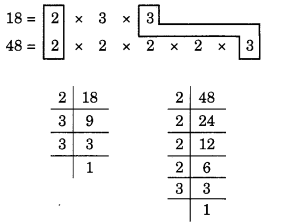Here, the common factors are 2 and 3.
Hence, the HCF = 2 x 3 = 6.

(b) The given numbers are 30 and 42.
Prime factorisations of 30 and 42, are: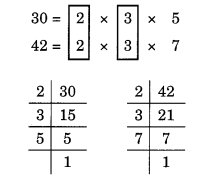Here, the common factors are 2 and 3.
Hence, the HCF = 2 x 3 = 6.

(c) Given numbers are 18 and 60.
Prime factorisations of 18 and 60 are: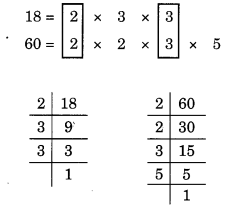Here, the common factors are 2 and 3.
Hence, the HCF of 18 and 60 = 2 x 3 = 6.

(d) Given numbers are 27 and 63.
Prime factorisations of 27 and 63 are:Here, the common factor is 3 (occurring twice).
Hence, the HCF = 3 x 3 = 9.

(e) Given numbers are 36 and 84.
Prime factorisations of 36 and 84 are:Here, the common factors are 2, 2 and 3.
Hence, the HCF = 2 x 2 x 3 = 12.

(f) Given numbers are 34 and 102.
Prime factorisations of 34 and 102 are: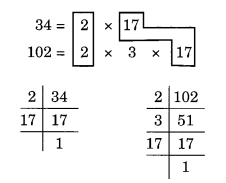Here, the common factors are 2 and 17.
Thus, HCF is 2 x 17 = 34.

(g) The given numbers are 70, 105 and 175.
Prime factorisatios of 70, 105 and 175 are: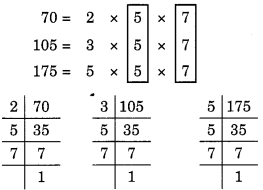Here, common factors are 5 and 7.
Hence, the HCF of 70, 105 and 175 is 5 x 7 = 35.

(h) Given numbers are 91, 112 and 49.
Prime factorisations of 91, 112 and 49 are:Here, the common factor is 7.
Hence, the HCF = 7.

(i) Given numbers are 18, 54 and 81.
Prime factorisations of 18, 54 and 81 are: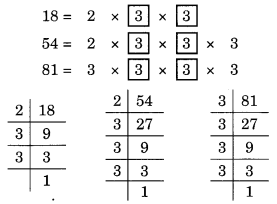Here, the common factor is 3 (occurring twice).
Thus, the HCF = 3 x 3 = 9.

(j) Given numbers are 12, 45 and 75.
Prime factorisations of 12, 45 and 75 are:Here, the common factor is 3.
Hence, the HCF = 3.

Ex 3.6 Class 6 Maths Question 2.
What is the HCF of two consecutive
(a) numbers?
(b) even numbers?
(c) odd numbers?
Solution:
(a) The common factor of two consecutive numbers is always 1.
Hence, the HCF = 1.
(b) The common factors of two consecutive even numbers are 1 and 2.
Hence, the HCF = 1 x 2 = 2.
(c) The common factor of two consecutive odd numbers is 1.
Hence, the HCF = 1.

Ex 3.6 Class 6 Maths Question 3.
HCF of co-prime numbers 4 and 15 was found as follows by factorisation:
4 = 2 x 2 and 15 = 3 x 15. Since there is no common prime factors, so HCF of 4 and 15 is 0.
Is the answer correct? If not, what is the correct HCF?
Solution:
Reason: 0 is not the prime factor of any number.
1 is always the prime factor of co-prime number.
Hence, the correct HCF of 4 and 15 is 1.

Move to Top → Playing with Numbers

### Playing with Numbers Class 6 Ex 3.7

Ex 3.7 Class 6 Maths Question 1.
Renu purchases two bags of fertiliser of weights 75 kg and 69 kg. Find the maximum value of weight which can measure the weight of the fertiliser exact number of times.
Solution:
Maximum value of weight which can measure the given weight exact number of time = HCF of 75 g and 69 kg
Prime factorisations of 75 and 69 areHere, the common factor is 3.
∴ HCF of 75 and 69 = 3.
Hence, the required maximum value of weight = 3 kg.

Ex 3.7 Class 6 Maths Question 2.
Three boys.step off together from the same spot. Their steps measure 63 cm, 70 cm and 77 cm respectively. What is the minimum distance each should cover so that all can cover the distance in complete steps?
Solution:
The minimum distance that each boy should walk must be the least common multiple (LCM) of the measure of their steps.
To find LCM of 63, 70 and 77, we use division method.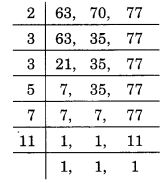∴ LCM of 63, 70 and 77 = 2 x 3 x 3 x 5 x 7 x 11 = 6930
Hence, the required minimum distance = 6930 cm.

Ex 3.7 Class 6 Maths Question 3.
The length, breadth and height of a room are 825 cm, 675 cm and 450 cm respectively. Find the longest tape which can measure the three dimensions of the room exactly.
Solution:
The longest tape required to measure the three dimensions of the room = HCF of 825, 675 and 450
Prime factorisations of 825, 675 and 450 are
825 = 3 x 5 x 5 x 11
675 = 3 x 3 x 3 x 5 x 5
450 = 2 x 3 x 3 x 5 x 5Here, common factors are 3, 5 (two times).
∴ HCF of 825, 675 and 450 = 3 x 5 x 5 = 75
Hence, the required longest tape = 75 cm.

Ex 3.7 Class 6 Maths Question 4.
Determine the smallest 3-digit number which is exactly divisible by 6, 8 and 12.
Solution:
The smallest 3-digit number = 100
Since LCM of 6, 8 and 12 is divisible by them.∴ LCM of 6, 8 and 12 = 2 x 2 x 2 x 3 = 24
Since, all the multiples of 24 will also be divisible by 6, 8 and 12.So, the smallest multiple of 24 in three digits will be just above
100 = (100 – 4) + 24 = 96 + 24 = 120
Hence, the required number is 120.

Ex 3.7 Class 6 Maths Question 5.
Determine the greatest 3-digit number exactly divisible by 8, 10 and 12.
Solution:
To find the LCM of 8, 10 and 12, we have∴ LCM of 8, 10 and 12 = 2 x 2 x 2 x 3 x 5 = 120
The greatest 3-digit number = 999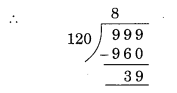∴ Multiple of 120 just below 999 is 960.
Hence, the required number is 960.

Ex 3.7 Class 6 Maths Question 6.
The traffic lights at three different road crossings change after every 48 seconds, 72 seconds and 108 seconds respectively. If they change simultaneously at 7 a.m., at what time will they change simultaneously again?
Solution:
To find the LCM of 48, 72 and 108, we have∴ LCM = 2 x 2 x 2 x 2 x 3 x 3 x 3= 432
So, after 432 seconds, the light will change simultaneously.
Hence, the required time = 432 seconds = 7 minutes 12 seconds i.e., 7 minutes 12 seconds past 7 a.m.

Ex 3.7 Class 6 Maths Question 7.
Three tankers contain 403 litres, 434 litres and 465 litres of diesel respectively. Find the maximum capacity of a container that can measure the diesel of the three containers exact number of times.
Solution:
Maximum capacity of the required measure is equal to the HCF of 403, 434 and 465.
Prime factorisations of 403, 434 and 465 areCommon factor = 31.
So, the HCF of 403, 434 and 465 = 31.
Hence, the maximum capacity of the required container = 31 litres.

Ex 3.7 Class 6 Maths Question 8.
Find the least number which when divided by 6, 15 and 18 leave remainder 5 in each case.
Solution:
To find the LCM of 6, 15 and 18, we have∴ LCM of 6, 15 and 18 = 2 x 3 x 3 x 5 = 90.
Here, 90 is the least number exactly divisible by 6, 15 and 18.
To get a remainder 5, the least number will be 90 + 5 = 95.
Hence, the required number is 95.

Ex 3.7 Class 6 Maths Question 9.
Find the smallest 4-digit number which is divisible by 18, 24 and 32.
Solution:
The smallest 4-digit number = 1000.
To find the LCM of 18, 24 and 32, we have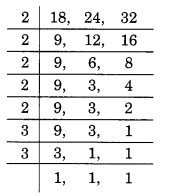∴ LCM = 2 x 2 x 2 x 2 x 2 x 3 x 3 = 288
Since, 288 is the smallest number which is exactly divisible by 18, 24 and 32.
But it is not a 4-digit number.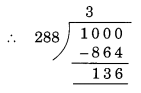So, the multiple of 288 just above 1000 is: 1000 – 136 + 288 = 1152.
Hence, the required number is 1152.

Ex 3.7 Class 6 Maths Question 10.
Find the LCM of the following numbers:
(a) 9 and 4
(b) 12 and 5
(c) 6 and 5
(d) 15 and 4
Observe a common property in the obtained ’ LCMs. Is LCM the product of two numbers in each case?
Solution:
(a) To find the LCM of 9 and 4, we have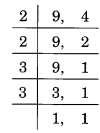∴ LCM = 2 x 2 x 3 x 3 = 36.
The product 9 and 4 = 9 x 4 = 36.
Hence, the LCM of 9 and 4 = Product of 9 and 4.

(b) To find LCM of 12 and 5, we have∴ LCM = 2 x 2 x 3 x 5 = 60.
The product of 12 and 5 = 12 x 5 = 60.
Hence, the LCM of 12 and 5 = Product of 12 and 5.

(c) To find the LCM of 6 and 5, we have∴ LCM = 2 x 3 x 5 = 30.
The product of 6 and 5 = 6 x 5 = 30.
Hence, the LCM of 6 and 5 = Product of 6 and 5.

(d) To find the LCM of 15 and 4, we have∴ LCM = 2 x 2 x 3 x 5 = 60.
Product of the numbers 15 and 4 = 15 x 4 = 60.
Hence, the LCM of 15 and 4 = Product of 15 and 4.

Ex 3.7 Class 6 Maths Question 11.
Find the LCM of the following numbers in which one number is the factor of the other.
(a) 5, 20
(b) 6, 18
(c) 12, 48
(d) 9, 45
What do you observe in the results obtained?
Solution:
(a) To find the LCM of 5 and 20, we have∴ LCM = 2 x 2 x 5 = 20.
Hence, the LCM of 5 and 20 = 20.

(b) To find the LCM of 6 and 18, we have∴ LCM = 2 x 3 x 3 = 18.
Hence, the LCM of 6 and 18 = 18.

(c) To find the LCM of 12 and 48, we haveLCM = 2 x 2 x 2 x 2 x 3 = 48.
Hence, the LCM of 12 and 48 = 48.

(d) To find the LCM of 9 and 45, we have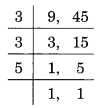∴ LCM = 3 x 3 x 5 = 45.
Hence, the LCM of 9 and 45 = 45.
From the above examples, we observe that the LCM of the two numbers, where one number is a factor of the other, is the greater number.

Move to Top → Playing with Numbers

CBSE Class 6 Study Material